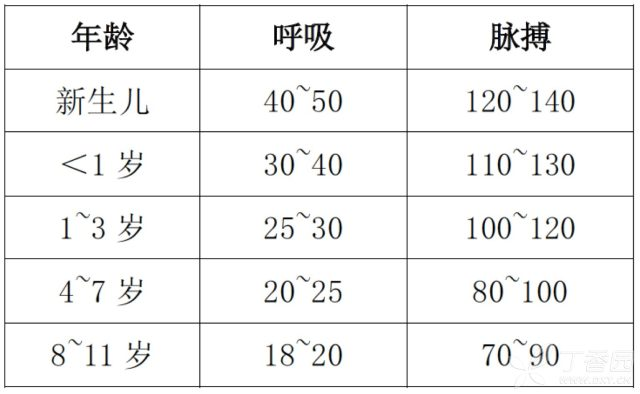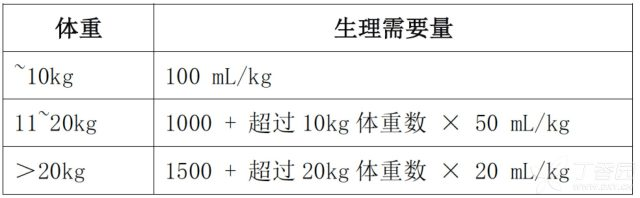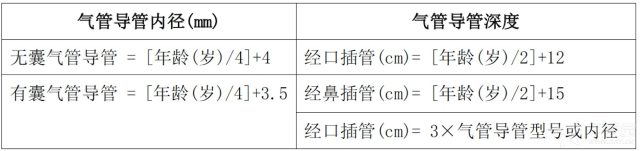# 一文掌握：13 类儿科常用计算公式

2017-07-08 19:20 来源：丁香园 作者：文侠

- | +

1. 尿量

2. 体重

• 2～6 岁：体重 (kg) = 年龄 (岁) × 2 + 8

• 7～10 岁：体重 (kg) = 年龄 (岁) × 3 + 2

3. 身高

2～10 岁身高 (cm) = (年龄 × 6.5) + 76

4. 胸围

5. 血压

• 收缩压 (mmHg) = 80 + (年龄 × 2）

• 舒张压 (mmHg) = 2/3 收缩压

6. 呼吸、脉搏7. 体表面积

• 体重 ≤ 30 Kg 儿童体表面积 (m2) = 体重 (kg) × 0.035 + 0.1

• 体重＞30 Kg 儿童体表面积 (m2) = [体重 (kg)－30] × 0.02 + 1.05

• 儿童体表面积 (m2) = 0.007184 × 身高 (cm) 0.725 × 体重 (kg)0.425

• 儿童体表面积 (m2) = 0.0061×身高 (cm) + 0.0128 × 体重 (kg) － 0.1529

8. 液体疗法

• 1 周内新生儿血浆渗透压 = 5 + 1.86 × 血清钠 (mmoL) + 2.8 × 尿素氮 (mg/dL) + 葡萄糖 (mmol) ÷ 18

• 5% 碳酸氢钠 (mL) = (-BE) × 0.5 × 体重 (kg)

• 5% 碳酸氢钠 (mL) = [ 22－实际碳酸氢盐 (mmol)] × 0.5 × 体重 (kg)

• 单纯失水型高钠血症需水量 (L) = 0.6 × 体重 (kg) × [ (患儿血清钠 mmol/L÷140) － 1 ]

• 低钠血症所需钠 mmol = [ 130－测得血钠 (mmol/L) ]×体重 (kg) × 0.3

• 所需 3% 氯化钠 (mL) = [ (125－血清钠) mmol/L × 0.6 × 体重 (kg) ] ÷ 0.5

9. 阴离子间隙

10. 生理需要量11. 泌尿系统

• 肾衰指数 = [尿钠 (mmol/L) × 血浆肌酐 (μmol/L) ] ÷ 尿肌酐 (μmol/L)

• 滤过钠排泄分数 = 尿钠 (mmol/L) × 血浆肌酐 (μmol/L) ÷ 血清钠 (mmol/L) 尿肌酐 (μmol/L)

• 内生肌酐清除率 (CCr) = 尿肌酐 (Cr) × 每分钟尿量 (mL/min) ÷ 血 Cr 浓度

• Schwartz 计算公式测算 CCr = 身高 (cm) ÷ 血 Cr (μmol/L) × K 值

• 矫正 GFR (肾小球率过滤) = 实际 GFR × 1.73 ÷ 小儿体表面积 (m2)

12. 2 岁以上气管导管内径和深度13. 微量泵静脉输液计算公式

(1) 乘 6 原则

• 药物输注速率单位为 mg 或 μg/kg·min

• 100 mL 液体药物剂量 (g 或者 mg)= [6×体重 (kg) × 药物输注速率 (mg 或 μg/kg·min)] ÷ 静脉输液速度 (mL/h)

(2) 乘 3 原则

• 药物输注速率单位为 mg 或 μg/kg·min

• 50 mL 液体药物剂量 (g 或者 mg) = [3×体重 (kg) × 药物输注速率 (mg 或 μg/kg·min)]÷静脉输液速度 (mL/h)

(3) 乘 0.1 原则

• 药物输注速率单位为 mg 或 μg/kg·h

• 100 mL 液体药物剂量 (g 或者 mg) = [6×体重 (kg) × 药物输注速率 (mg 或 μg/kg.h)] ÷ [ 60 × 静脉输液速度 (mL/h)]

(4) 乘 0.05 原则

• 药物输注速率单位为 mg 或 μg/kg·h

• 50 mL 液体药物剂量 (g 或者 mg) =[3×体重 (kg) × 药物输注速率 (mg 或 μg/kg.h)] ÷ [60 × 静脉输液速度 (mL/h)]

(5) 需加入某容量液体的药物剂量 (μg) = [60 × 液体容量 (mL) × 体重 (kg) × 药物输注速率 (μg/kg.min)] ÷ 输液速度 (mL/h)

(6) 需加入某容量液体的药物剂量 (μg) = [液体容量 (mL) × 体重 (kg) × 药物输注速率 (μg/kg·h) ] ÷ 输液速度 (mL/h)

os
os
os

os
os
os

os
os
os
• App下载